# Why is there a minimum output rate?

The micro-controller performs tasks one after the other. It must complete the sampling of all configured channels before sending another serial output out.

The example below show the task scheduling where 3 channels are computed. The output rate must be larger than the time it takes to sample all channels.

# What happen if the output rate is too low

If a low output rate is set the microcontroller will respect what is configured and output serial data at the given rate. The output stream will show duplicate values in subsequent output.

Take a look at the example below with a RPICT8 card sampled at 2 seconds where the minimum output rate is 3.2 seconds.
Some of the duplicated values are circled in red.

In this case because the micro-controller is not able to sample all channels it outputs the result in memory twice for some fields.

# Calculating the minimum output rate

The minimum output rate is given by

Where

• Ntasks is the number of channels or the number of node the mcu has to compute.
• Ncycle is the number of cycles used to compute the RMS value.
• Frequency is the expected frequency of the signal. (This is usually 50 or 60Hz).

Some examples

• RPICT8 by default has 8 channels. Ncycle = 20. For 50Hz the minimum output rate is 3.2 seconds. (2.7s for 60Hz)
• RPICT7V1 by default has 7 channels. Ncycle = 20. For 50Hz the minimum output rate is 2.8 seconds. (2.3s for 60Hz)
• RPICT4V3 by default has 4 channels. Ncycle = 20. For 50Hz the minimum output rate is 1.6 seconds. (1.3s for 60Hz)
• RPICT3V1 and RPICT3T1 have 3 channels. Ncycle = 20. For 50Hz the minimum output rate is 1.2 seconds. (1.0s for 60Hz)

# How to achieve lower output rate?

Reducing the minimum output rate means reducing Ntasks or Ncycle or both.

## Reducing Ncycle

This is probably the must accessible approach.

Ncycle is the number of cycles used to compute the RMS value. This can be considered like an averaging window. Reducing Ncycle will have the same effect has reducing an averaging window.

By default Ncycle is set to 20 cycles.
We would not recommend to use smaller Ncycle than 5 cycles. Although Ncycle=1 can be achieved but you might have to perform some tests to deem the result acceptable.

There are 2 ways to change the Ncycle parameter.

### Command line method

Dump the config with

```lcl-rpict-config.py -a
```

Open the config file

```nano /tmp/rpict.conf
```

Find the line that say Ncycle = 20 in the file.
Modify it to your need then save and exit. Reload the config in the device

```lcl-rpict-config.py -a -w /tmp/rpict.conf
```

### Web Tool method

Run the web tool on the Raspberrypi
RPICT_configuration_web_tool#Run_the_server

Go to the "Full Configuration" page.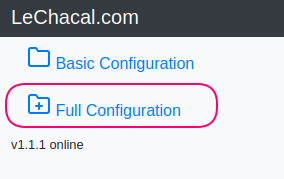Use this icon to load the config from the device.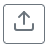Change the Ncycle parameter indicated below.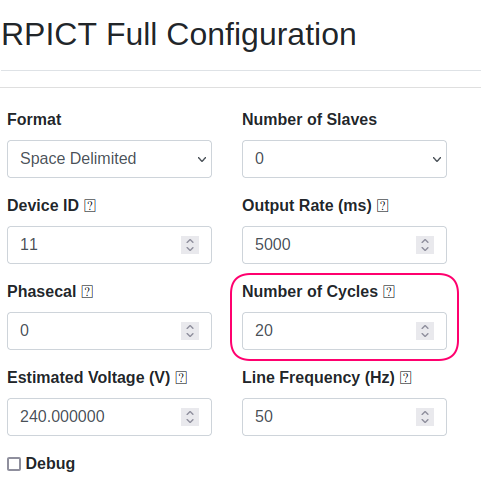Look at the "Summary" section to see the effect on the minimum output rate.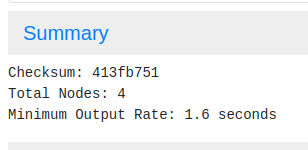Load the config in the device with this icon.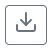This is not always possible especially if you need to use all channels on the RPICT card.

However it worth considering removing unused channels as this would make the mcu running less tasks and allow a lower output.

To remove unwanted channels go to "Full configuration" page in the web tool.
RPICT_configuration_web_tool#Run_the_server

You must then delete the channels in both the "Output Channels" section and the "Node" section.

# Going away from the problem entirely

The issue with duplicate values might not always be a problem for some applications. This might probably not affect your application down the line. You would need to decide if that's the case or not. However in general we would recommend setting the appropriate output rate for best results.

A way to get around the issue is to use the full output firmware. The output method here is very different as each channel is given in serial as soon as it has finished computing.